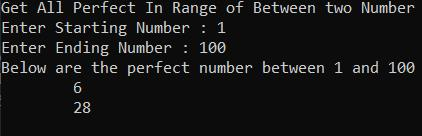Loading, please wait...# Get All Perfect Numbers In A Range Of Two Numbers

Jan 13, 2021 c# , perfect number , , 2519 Views
In this article we will create a C# program for finding all perfect numbers between two numbers. Here we will get two inputs from user and then perform an operation on it.

## Get All Perfect Numbers In A Range Of Two Numbers

In this article, we will create a C# program for finding all perfect numbers between two numbers. Here we will get two inputs from the user and then perform an operation on it.

Also, check out:
In the above blog, I had covered what is the perfect number and how to check if any number is perfect or not .

Program to find all perfect numbers in the range:

``````using System;
using System.Collections.Generic;
using System.Linq;
using System.Text;

namespace PerfectNumberInRange
{
class Program
{
static void Main(string[] args)
{
int startingNumber, endingNumbeer;
Console.WriteLine("Get All Perfect In Range of Between two Number");
Console.Write("Enter Starting Number : ");
Console.Write("Enter Ending Number : ");

Console.WriteLine("Below are the perfect number between " + startingNumber + " and " + endingNumbeer);

for (int i = startingNumber; i <= endingNumbeer; i++)
{
decimal sum = 0;
for (int j = 1; j < i; j++)
{
if (i % j == 0)
sum = sum + j;
}
if (sum == i)
Console.WriteLine("\t" +i);
}

}
}
}  ``````

OutputExplanation
• Here we get two inputs from the user, a starting number, and an ending number.
• Then we iterate the loop from the starting number to the ending number.
• In this loop iterate another loop from 1 to the value of the parent loop and declare a new variable called sum and assign zero to it.
• In child loop we modulate the parent loop value with the child loop value. If the value is equal to zero then add that value in the sum variable.
• After the child loop completes, check if the sum value and parent loop value both are equal or not. If both values are equal then this number is a perfect number.
• Then we use the Console.ReadKey() method to read any key from the console. By entering this line of code, the program will wait and not exit immediately. The program will wait for the user to enter any key before finally exiting. If you don't include this statement in the code, the program will exit as soon as it is run.
I hope you find this blog helpful. If you get any help from this blog please share it with your friends